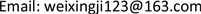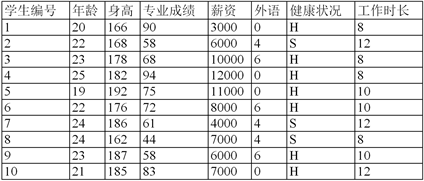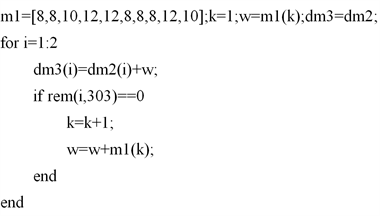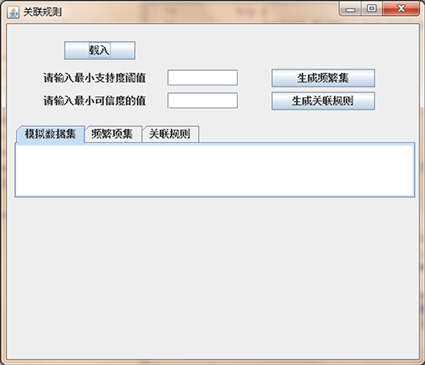﻿ 基于Apriori算法的毕业生需求状况的可视化分析和预测 Visual Analysis and Prediction of Graduate Demand Based on Apriori Algorithm

Artificial Intelligence and Robotics Research
Vol. 08  No. 02 ( 2019 ), Article ID: 30079 , 6 pages
10.12677/AIRR.2019.82008

Visual Analysis and Prediction of Graduate Demand Based on Apriori Algorithm

Qingzhen Wang, Xiao Ju

Department of Information Engineering, Zhengzhou University of Science and Technology, Zhengzhou HenanReceived: Apr. 8th, 2019; accepted: Apr. 29th, 2019; published: May 6th, 2019ABSTRACT

In order to quickly establish a good supply and demand relationship between enterprises and college students, in the general environment of increasing graduates’ employment difficulties, we are using a network platform to collect information on the basic supply and demand of graduates and the data collected for visual analysis and prediction research. Firstly, the data is extracted from the network platform, and Excel is used to sort out the graph. Then the task of data mining is determined; the data of chart is preprocessed; and the data is analyzed based on Apriori algorithm. Finally, the analysis and prediction are completed by Java program.1. 引言

2. 研究概述

2.1. 收集数据Figure 1. Information thumbnails for graduatesFigure 2. Thumbnail of enterprise demand information

2.2. 分析呈现数据

1、[18,20]1，[21,23]=2，[24,26]=3

2、[0,33]=1，[34,66]=2，[67,100]=3

3、[0,3000]=1，[3000,4500]=2，[4500,6000]=3

4、=1，=2，=3

5、[0,8]=1，[8,10]=2，[10,12]=32.3. 可视化分析预测

Apriori算法  是常用的用于挖掘出数据关联规则的算法，它用来找出数据值中频繁出现的数据集合，找出这些集合的模式有助于我们做一些决策。常用的频繁项集的评估标准有支持度，置信度和提升度三个。

$\text{Support}\left(\text{X},\text{Y}\right)=\text{P}\left(\text{X},\text{Y}\right)=\text{number}\left(\text{XY}\right)/\text{num}\left(\text{AllSamples}\right)$ (1)

$\text{Support}\left(\text{X},\text{Y},\text{Z}\right)=\text{P}\left(\text{X},\text{Y},\text{Z}\right)=\text{number}\left(\text{XYZ}\right)/\text{num}\left(\text{AllSamples}\right)$ (2)

$\text{Confidence}\left(\text{X}⇐\text{Y}\right)=\text{P}\left(\text{Z}|\text{Y}\right)=\text{P}\left(\text{XY}\right)/\text{P}\left(\text{Y}\right)$ (3)

$\text{Confidence}\left(\text{X}⇐\text{YZ}\right)=\text{P}\left(\text{X}|\text{YZ}\right)=\text{P}\left(\text{XYZ}\right)/\text{P}\left(\text{YZ}\right)$ (4)

$\text{Lift}\left(\text{X}⇐\text{Y}\right)=\text{P}\left(\text{X}|\text{Y}\right)/\text{P}\left(\text{X}\right)=\text{Confidence}\left(\text{X}⇐\text{Y}\right)/\text{P}\left(\text{X}\right)$ (5)Figure 3. Main interface of association rules

3. 结束语

985高校，六级，农村 7~10 k，一线城市

985高校，六级，城市 5~7 k，二线城市

1) 985高校毕业生对薪资要求较高，更倾向于一线城市

2) 专业不是影响就业的主要因素

3) 城市生源的毕业生倾向于二线城市，对薪资最低接受限度较低

1) 五百强企业主要分布在一线及海外城市，且工资普遍较高。最看重毕业生的条件是毕业院校和外语水平，要求偏高。

2) 国企和私企大多分布在二线和一线城市，对毕业生的要求院校依旧偏高，但不高于五百强企业，对外语水平的要求比较宽松。

3) 家庭条件不是影响就业的主要因素。

1) 毕业生和企业在高校、外语等方面的供需关系是比较平衡的。

2) 毕业生和企业在薪资方面有一定矛盾。毕业生需求较高，企业提供的较低。

2018年度河南省大中专院校就业创业研究立项课题JYB2018074。

Visual Analysis and Prediction of Graduate Demand Based on Apriori Algorithm[J]. 人工智能与机器人研究, 2019, 08(02): 62-67. https://doi.org/10.12677/AIRR.2019.82008

1. 1. 常大俊. 基于数据仓库和OLAP的决策技术研究[D]: [硕士学位论文]. 长春: 长春理工大学, 2009.

2. 2. Shih, Y.S. (1999) Families of Splitting Criteria for Classification Trees. Statistics and Computing.

3. 3. 韩天鹏, 宋中山. Apriori算法的改进[J]. 电脑知识与技术(学术交流), 2007.

4. 4. 谢俏丽. 基于组合预测模型的湖北省卫生人力资源需求预测研究[D]: [硕士学位论文]. 武汉: 华中科技大学, 2016.

5. 5. 陈必坤, 赵蓉英. 学科知识可视化分析的理伭研究[J]. 情报理论与实践, 2015, 38(1): 23-29.